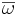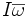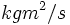# Angular momentum

## Definition

### For a single body

The angular momentum of a body with angular velocity equal to the vector$\overline{\omega}$ and moment of inertia$I$ about the axis of rotation is the vector$I \overline{\omega}$.

## Units and dimensions

MLT dimensions$ML^2T^{-1}$: MLT;1;2;-1
SI units$J-s$ (Joule-second) or$kgm^2/s$ (kilogram meter squared per second)